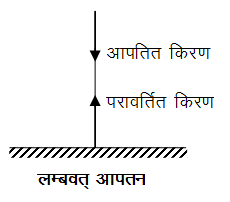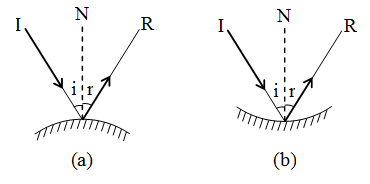Ex﻿

# 3. Light Reflection

#### Laws Of Reflection Of Light

Laws Of Reflection Of Light
First law : The incident ray, the reflected ray and the normal at the point of incidence, all lie in the same plane.
Second law : The angle of reflection (∠ r) is always equal to the angle of incidence (∠ i).
i.e., ∠ r = ∠ i
(For normal incidence, i = 0, r = 0. The ray is reflected back along normal).
(i) A ray of light striking the surface normally retraces its path.
When a ray of light strikes a surface normally, then angle of incidence is zero i.e., ∠ i = 0. According to the law of reflection, ∠ r = ∠ i,  ∠ r = 0 i.e. the reflected ray is also perpendicular to the surface. Thus, an incident ray normal to the surface (i.e. perpendicular to the surface) retraces its path as shown in figure.(ii) Laws of reflection are also obeyed when light is reflected from the spherical or curved surfaces as shown in figure (a) and (b)( Reflection from curved surface)

If you want to give information about online courses to other students, then share it with more and more on Facebook, Twitter, Google Plus. The more the shares will be, the more students will benefit. The share buttons are given below for your convenience.
×

#### NTSE Physics (Class X)

• Electricity
• Magnetic Effect of Curent
• Light Reflection
• Light Refraction
• Human Eye & Colourful World
• Source of Energy
• Motion

#### NTSE Physics (Class IX)

• Force & laws of motion
• Work and Energy
• Gravitation
• Sound

SHOW CHAPTERS